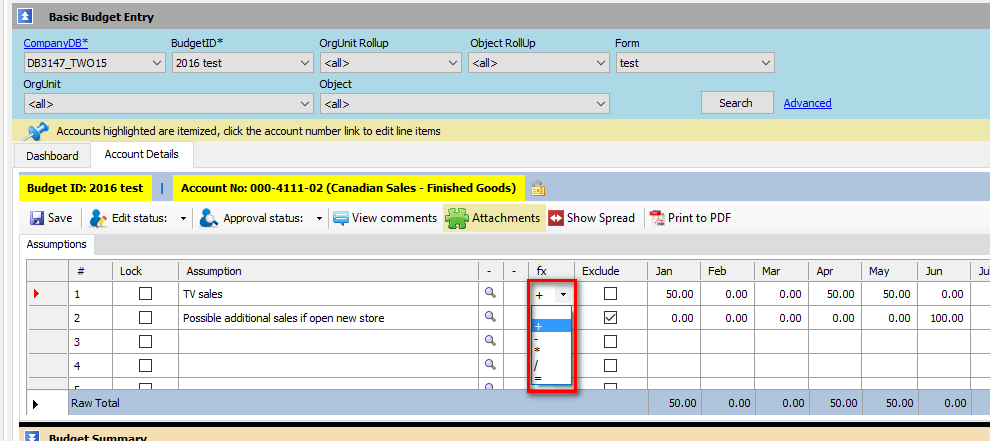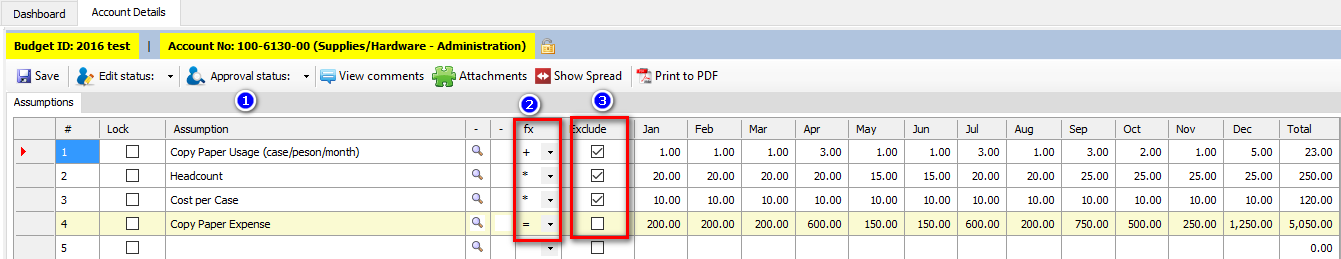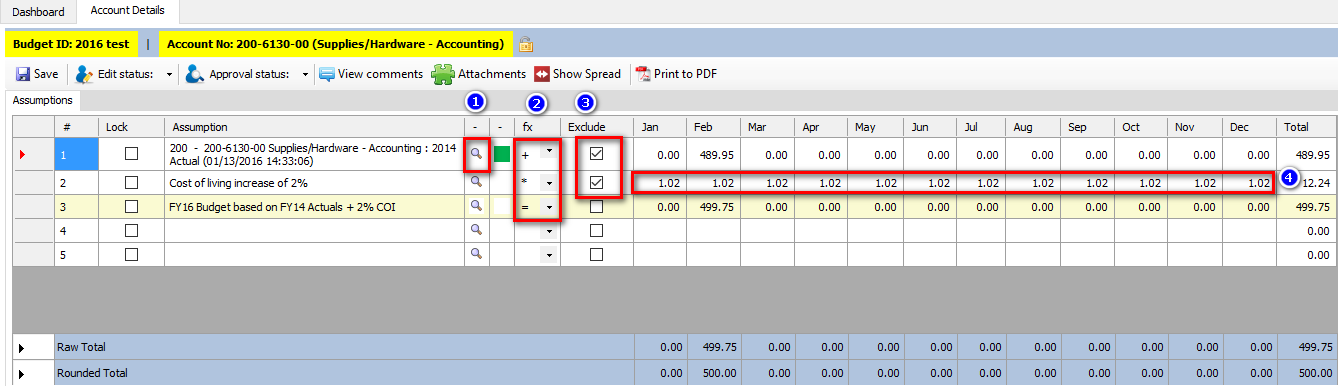This standard functionality is available on a number of screens including Basic Budget Entry/Account Details and Import Budget Assumptions.

Please note that this functionality can be used in conjunction with the Exclude Row Function and the Lookup function.

This functionality allows data in rows to be added together, divided, multiplied, subtracted and then totaled.  These math functions are accessed by clicking on the pull down menu in the row that you wish to add a function to.   In this case the data in row 1 will be added to the total.Below are examples of how this functionality can be used:

A) Supporting Details

The below examples adds rows 1 and 2 together and each row provides the timing, description and amount  of vehicle insurance expense by vehicle.B) Calculations using Exclude Function

The below example is calculating the Copy Paper Expense taking into account the monthly usage per person per month, the Headcount and cost per case.

1) Enter description assumptions;

2) Enter in the math functions required; and

3) Exclude rows that should not be added to the Grand Total.  Please note only the row with the "=" and with the Exclude that is unchecked will be part of the total expense.C) Calculations using Lookup and Exclude Functions

In this example we are using the Lookup function to pull the actual data from FY14.  We are using this as a base amount and then increasing it by a 2% cost of living expense (inflationary factor) to result in the FY16 Budget.

1) Use the Lookup functionality to select the data you wish to use;

2) Enter in the math functions required;

3) Exclude rows that should be excluded from the total; and

4) Enter in the cost of living (inflationary) factor that you wish to increase the expense by.Please note there can be more than one calculation on an entry sheet as only rows that are not excluded will be included in the Total.